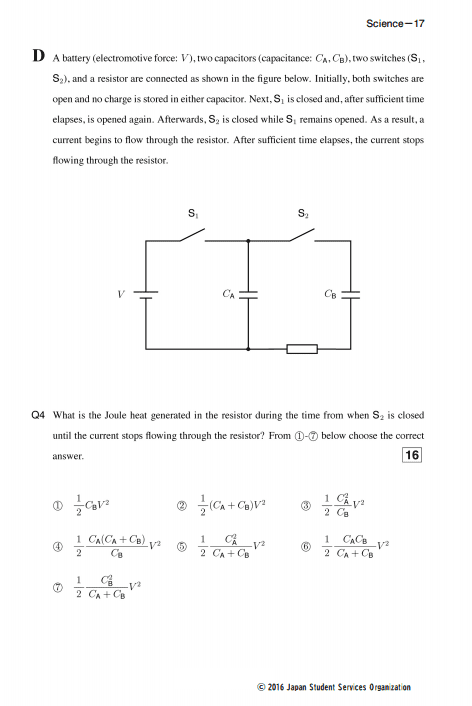# A circuit with switches, capacitators and resistors

Homework Statement:
A battery(electromotive force: V), two capacitors (capacitance: Ca,Cb), two switches (S1, S2), and a resistor are connect as shown in figure below. Initially, both switches are open and no charge in stored in either capacitor. Next, S1 is closed and, after sufficiente time elapses, is opened again. Afterwards, S2, is closed while S1 remains opened. As a result, a current begins to flow through the resistor. After sufficient time elapses the current stops flowing through the resistor. What is the Joule heat generated in the resistor during the time from when S2 is closed until the current stops flowing through the resistor?
Relevant Equations:
Q = C.V
Epe = QV/2
V = R.i
P = V.i
q'a + qb = qaTo solve this question first I calculated the potential energy the capacitor A stored. It's equal a: Ca.V²/2. Ok, so when switch S1 is open and S2 is closed I calculated the equivalent capacitance as if they were in series --> 1/Ceq = 1/Ca + 1/Cb --> Ceq = (Ca.Cb)/(Ca+Cb). So I used the formula: CeqV²/2 --> (Ca.Cb.V²)/2(Ca+Cb) and I found the awnser. The problem is, my friend said that the capacitors are in parallel, but I solved by series, who is right? Which is the best way to solve this question? Thanks

## Answers and Replies

TSny
Homework Helper
Gold Member
I calculated the equivalent capacitance as if they were in series --> 1/Ceq = 1/Ca + 1/Cb --> Ceq = (Ca.Cb)/(Ca+Cb). So I used the formula: CeqV²/2 --> (Ca.Cb.V²)/2(Ca+Cb)
This is not a correct calculation. Even if the calculation were correct, wouldn't your result represent the final energy stored in the two capacitors? But that's not what you are asked to find.

When you close the second switch, current will flow until some final condition is met. What is this final condition?

•eyespy
This is not a correct calculation. Even if the calculation were correct, wouldn't your result represent the final energy stored in the two capacitors? But that's not what you are asked to find.

When you close the second switch, current will flow until some final condition is met. What is this final condition?
It is when the voltage is equal in Capacitor A and B. They are in parallel or in serie?

.
It is when the voltage is equal in Capacitor A and B. They are in parallel or in serie?
Yes :)

In the situation where the current has stopped, there's no more voltage across R, so you could replace it by a wire. The capacitors will then both be in series and in parallel, and you will be able to use rules for both series and parallel circuits, and you should get the same result.
After computing the final voltage or charge on the capacitors, you'll need to do some more work to compute the energy dissipation in R from the loss in electric energy.

•eyespy
TSny
Homework Helper
Gold Member
It is when the voltage is equal in Capacitor A and B.
Yes.

Don't worry about whether or not the capacitors are in series or parallel. Try to picture what's going on physically with the charge. Before closing S2, there is a certain amount of positive charge on the upper plate of C1 and no charge on the upper plate of C2. After S2 is closed, how is the charge distributed? How does the total final charge on the upper plates compare to the initial charge on the upper plate of C1?

•eyespy
.

Yes :)

In the situation where the current has stopped, there's no more voltage across R, so you could replace it by a wire. The capacitors will then both be in series and in parallel, and you will be able to use rules for both series and parallel circuits, and you should get the same result.
After computing the final voltage or charge on the capacitors, you'll need to do some more work to compute the energy dissipation in R from the loss in electric energy.
Yes.

Don't worry about whether or not the capacitors are in series or parallel. Try to picture what's going on physically with the charge. Before closing S2, there is a certain amount of positive charge on the upper plate of C1 and no charge on the upper plate of C2. After S2 is closed, how is the charge distributed? How does the total final charge on the upper plates compare to the initial charge on the upper plate of C1?
So, I will calculate q'a + qb = qa = CaVa--> q'a/Ca - qb/Cb = 0 --> q'a/Ca = qb/Cb. So CaV - q'a = q'aCb/Ca, manipulating q'a = Ca²V/(Ca+Cb). And I found that q'b = VCaCb/(Ca+Cb). Now what I have to do? I was making this exercise for more than one day lol

TSny
Homework Helper
Gold Member
So, I will calculate q'a + qb = qa = CaVa--> q'a/Ca - qb/Cb = 0 --> q'a/Ca = qb/Cb. So CaV - q'a = q'aCb/Ca, manipulating q'a = Ca²V/(Ca+Cb). And I found that q'b = VCaCb/(Ca+Cb). Now what I have to do?

Looks good. You now know the final charge on each capacitor. You can easily find the final V of each.

You should be able to find expressions for the final energy of each capacitor and compare to the initial energy in Ca.

Last edited: